#Function Repository Resource:

# NResidue

Find a numerical approximation of a residue of an expression around the specified point

Contributed by: Wolfram Research
 ResourceFunction["NResidue"][expr,{z,z0}] numerically finds the residue of expr near the point z=z0.

## Details and Options

The expression expr must be numeric when its argument x is numeric.
The residue is defined as the coefficient of (z-z0)-1 in the Laurent expansion of expr.
ResourceFunction["NResidue"] numerically integrates around a small circle centered at the point z0 in the complex plane. ResourceFunction["NResidue"] will return an incorrect result when the punctured disk is not analytic.
ResourceFunction["NResidue"] is unable to recognize small numbers that should in fact be zero. Chop is often needed to eliminate these spurious residuals.
Although Residue usually needs to be able to evaluate power series at a point, ResourceFunction["NResidue"] can find residues even if the power series cannot be computed.
ResourceFunction["NResidue"] has the same options as NIntegrate, with the following additions and changes:
 "Radius" 1/100 radius of contour on which integral is evaluated Method Trapezoidal integration method to use

## Examples

### Basic Examples

Residue of the function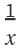about the origin:

 In:=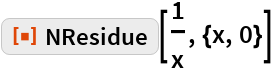Out=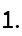### Scope

NResidue can find residues of functions with essential singularities:

 In:=Out=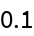Since Series is unable to handle essential singularities, Residue returns unevaluated:

 In:=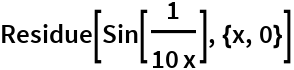Out=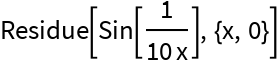NResidue allows for some error in the location of the pole:

 In:=Out=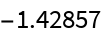Due to machine-precision arithmetic, z 1. is not a pole:

 In:=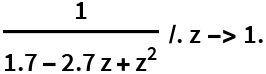Out=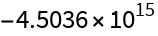With Residue, the error in the location of the pole yields a result of zero:

 In:=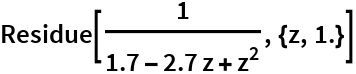Out=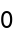### Generalizations & Extensions

NResidue threads element-wise over lists:

 In:=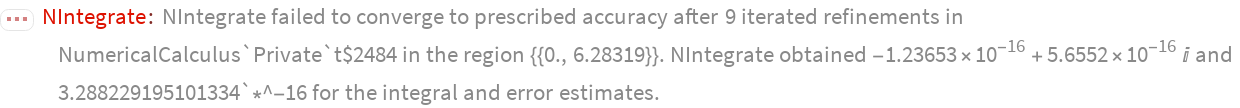Out=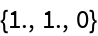### Options

#### Radius

Use "Radius" to shrink the radius of the contour of integration to isolate a single pole:

 In:=Out=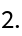In:=Out=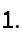Increase the radius to improve convergence of the integration if no other poles are nearby:

 In:=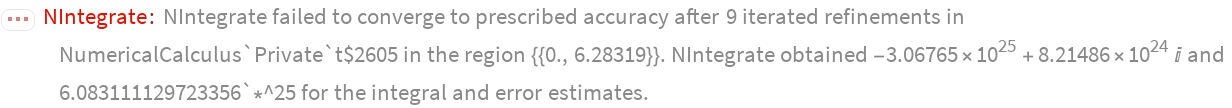Out=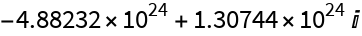In:=Out=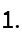#### WorkingPrecision

NResidue accepts options for NIntegrate, which are sometimes necessary to get an accurate result:

 In:=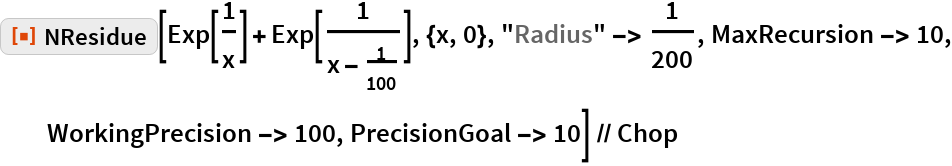Out=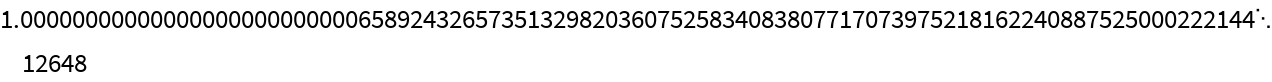### Applications

Use NResidue to evaluate derivatives of a function evaluated at a point:

 In:=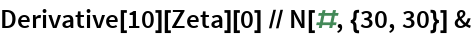Out=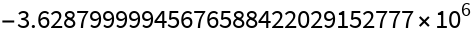In:=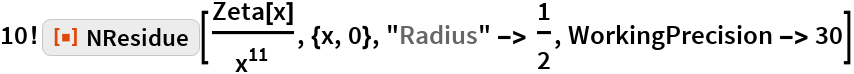Out=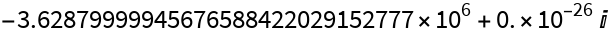Residues of numerical functions:

 In:=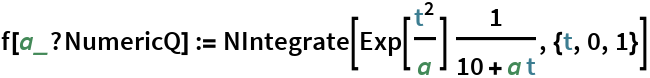In:=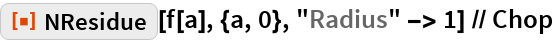Out=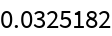### Properties & Relations

NSeries can also compute residues of numerical functions:

 In:=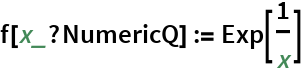In:=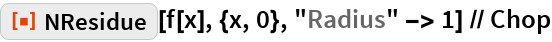Out=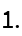Using NSeries:

 In:=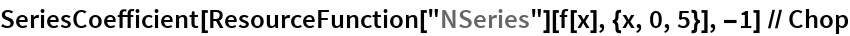Out=### Possible Issues

NResidue will return an incorrect result when the integration contour contains branch cuts:

 In:=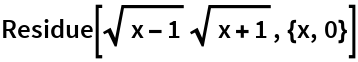Out=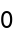In:=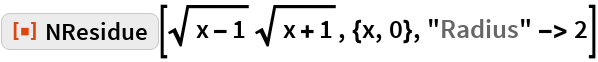Out=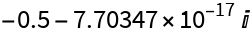## Requirements

Wolfram Language 11.3 (March 2018) or above